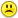+0

# Find the equivalent expression to the complex number (1+i)23+(1−i)^2 3.

0
999
2

Find the equivalent expression to the complex number (1+i)23+(1i)^3.

Aug 27, 2015

#2
+5

I don't know what you mean eitherAug 27, 2015

#1
+5

Find the equivalent expression to the complex number (1+i)23+(1−i)^2 3.

Do you mean (1+i23) + (1-i23)????????????

If that's what you meant, then the answer is=2

Aug 27, 2015
#2
+5
I don't know what you mean either